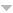## Publications

Export 28 results:
Sort by: Author Title Type [ Year]
2003
Cipriano, F. "A Stochastic variational principle for Burgers equation and its symmetries." STOCHASTIC ANALYSIS AND MATHEMATICAL PHYSICS II. Ed. R. } {Rebolledo. {TRENDS IN MATHEMATICS}. VIADUKSTRASSE 40-44, PO BOX 133, CH-4010 BASEL, SWITZERLAND: Catedra Presiden Analis Cualitat Sistemas Dinam Cuant; Univ Catol, Direcc Invest Postgrado; FONDECYT; ICCTICONICYT Exchange Programme, 2003. {29-46}. Abstract

{A stochastic variational principle for the classical Burgers equation is established. A solution of this equation can be considered as the velocity field of a stochastic process which is a minimum of an energy functional. A family of stochastic constants of the motion, determined in terms of the probability distribution of that process, yields the complete list of symmetries of the Burgers equation.}

1999
Cipriano, F. "The two dimensional Euler equation: A statistical study." COMMUNICATIONS IN MATHEMATICAL PHYSICS. 201 (1999): 139-154. Abstract

{We construct surface type measures on the level sets for the renormalized energy, which is an invariant quantity for the two dimensional periodic Euler flow, and prove the existence of weak solutions living on such level sets. Other classes of invariant measures for the motion are also introduced.}

Cipriano, F., and A. B. Cruzeiro. "Flows associated to tangent processes on the Wiener space." JOURNAL OF FUNCTIONAL ANALYSIS. 166 (1999): 310-331. Abstract

{We prove, under certain regularity assumptions on the coefficients, that tangent processes (namely semimartingales d xi(tau) = a dx(tau) + b d tau where a is an antisymmetric matrix) generate flows on the classical Wiener space. Main applications of the result can be found in the study of the geometry of path spaces. (C) 1999 Academic Press.}